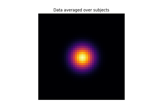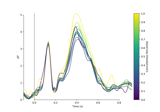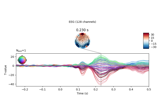# mne.stats.linear_regression¶

mne.stats.linear_regression(inst, design_matrix, names=None)[source]

Fit Ordinary Least Squares (OLS) regression.

Parameters
instinstance of `Epochs` | iterable of `SourceEstimate`

The data to be regressed. Contains all the trials, sensors, and time points for the regression. For Source Estimates, accepts either a list or a generator object.

design_matrix`ndarray`, shape (n_observations, n_regressors)

The regressors to be used. Must be a 2d array with as many rows as the first dimension of the data. The first column of this matrix will typically consist of ones (intercept column).

names

Optional parameter to name the regressors (i.e., the columns in the design matrix). If provided, the length must correspond to the number of columns present in design matrix (including the intercept, if present). Otherwise, the default names are `'x0'`, `'x1'`, `'x2', …, 'x(n-1)'` for `n` regressors.

Returns
results

For each regressor (key), a namedtuple is provided with the following attributes:

• `beta` : regression coefficients

• `stderr` : standard error of regression coefficients

• `t_val` : t statistics (`beta` / `stderr`)

• `p_val` : two-sided p-value of t statistic under the t distribution

• `mlog10_p_val` : -log₁₀-transformed p-value.

The tuple members are numpy arrays. The shape of each numpy array is the shape of the data minus the first dimension; e.g., if the shape of the original data was `(n_observations, n_channels, n_timepoints)`, then the shape of each of the arrays will be `(n_channels, n_timepoints)`.

## Examples using `mne.stats.linear_regression`¶